# Parallelogram, triangles etc

The area of a parallelogram is determined by multiplying the base, b, with the height, h, of the parallelogram: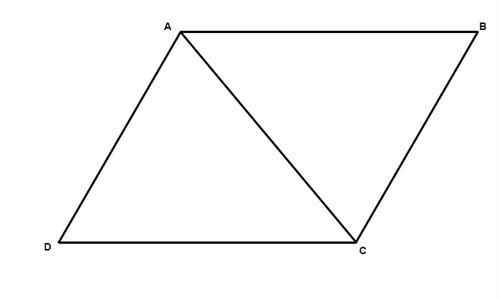$A=b\cdot h$

The area of a triangle is determined by multiplying the base, b, with the height, h, of the triangle and divide by two: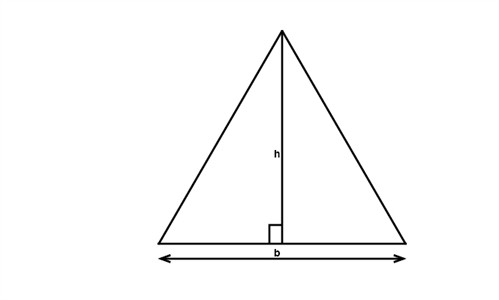$A=\frac{b\cdot h}{2}$

The area of a trapezoid is determined by multiplying the mean value of the two bases, b1 and b2, with the height, h, of the trapezoid: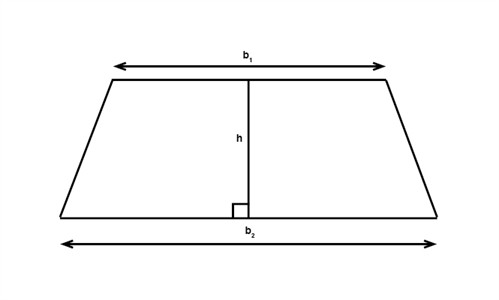$A=h\cdot \frac{b_{1}+b_{2}}{2}$

The area of a rhombus is determined by half the product of the two diagonals: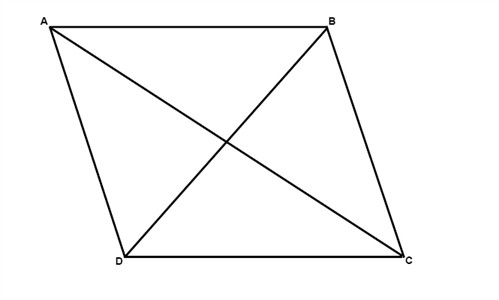$A=\frac{1}{2}(AC)(BD)$

The area of a regular polygon is determined by the product of the perimeter, P, and what is called an apothem, a (see figure below):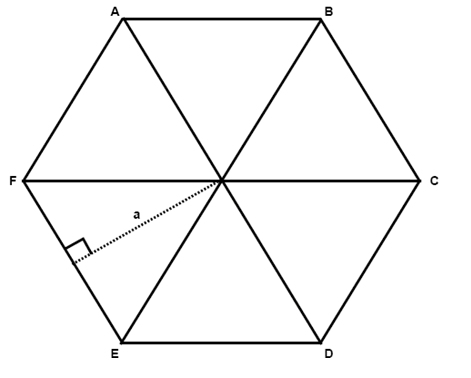$A=\frac{Pa}{2}$

The area of a circle is determined by multiplying the square of the radius, r, with the constant π, pi: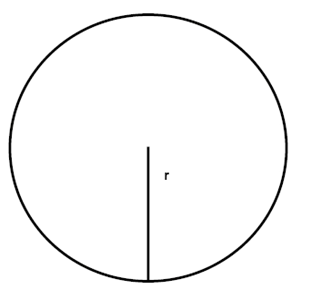$A=\pi r^{2}$

## Video lesson

Find the area of the triangle